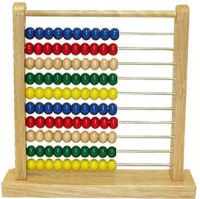# Top 10 mathematics programsFor many, it’s getting towards that fateful time of year again where exams are looming. If you’re a student and are busy swotting (or at least, you’re supposed to be) for your maths finals then take a trip to Softonic’s Mathematics section, where you could find the program that makes the difference between getting an A or a B.

There’s a multitude of helpful software on here, from simple chart plotters, such as the delightful Graph, to heavyweight apps that provide tools to solve complex equations. The number one application in this category Derive, is actually one of the most heavily-downloaded programs on Softonic. It helps you to get around tricky numerical and symbolic problems with the minimum of fuss, and is useful for a wide range of mathematical disciplines, including arithmetic, algebra, trigonometry, and calculus.

1. Derive Powerful system for doing symbolic and numeric mathematics
2. GraphDraw mathematical graphs in a coordinate system
3. Deadline Solve equations and plot graphs
4. Geometry Calculator Calculate and convert various geometrical values
5. MathWriter Write mathematical, scientific and engineering equations
6. Basic Facts Worksheet Factory – Sharpen your times-tables skills
7. Math Practice Demonstrate your great mathskills with this free software
8. Equation GrapherDraw and analyse mathematical functions
9. Math Calculator Calculate expression, derivative, root, extremum, and integral
10. 3DField Convert your data into contour maps and surface plots